Function Repository Resource:

# WaterOpticalConstants

Obtain optical constants of water valid from ultraviolet to microwave range

Contributed by: Julián Laverde
 ResourceFunction["WaterOpticalConstants"][] returns the optical constants of water at T=23°C and P=1bar for the wavelength of 600 nm. ResourceFunction["WaterOpticalConstants"][assoc] returns the optical constants of water for the conditions specified in the Association assoc.

## Details and Options

In ResourceFunction["WaterOpticalConstants"][assoc], the Association assoc can include the following elements:
 "Wavelength" 600 nm incident light wavelength "Temperature 23°C temperature of the water "Pressure" 1 bar pressure of the water
ResourceFunction["WaterOpticalConstants"] returns the optical constants of water in liquid or ice (Ih) phase, corresponding to the selected temperature and pressure at the given wavelength.
The available optical constants are the refractive index and the absorption coefficient.
The refractive index is the ratio between the speed of light in medium to speed in a vacuum.
The absorption coefficient, also called the attenuation coefficient or extinction coefficient, is a representative term for determining how far the incident light of a certain wavelength penetrates a material before being absorbed. The corresponding unit is in reciprocal meters.
The wavelength can be any quantity whose physical dimension is "LengthUnit". If given only as a number, the default unit of wavelength is nanometers. If unspecified, wavelength is taken by default to be 600nm. The valid range ofwavelength is from 200nm to 1.0 x 109nm for the absorption coefficient and 10nm to 1.0 x1010nm for the refractive index.
The temperature can be any quantity whose physical dimension is "TemperatureUnit". If given only as a number, the default unit of temperature is degrees Celsius. If unspecified, temperature is taken by default to be 23°C. The maximum value of temperature is 500°C.
The value of pressure can be any quantity whose physical dimension is "PressureUnit". If given only as a number, the default unit of pressure is bars. If unspecified, pressure is taken by default to be 1 bar.
The valid range of temperature and pressure corresponds to conditions in which water exists in liquid or solid (Ih) phase.

## Examples

### Basic Examples (3)

Use the default values:

 In:=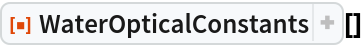Out=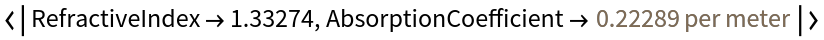Find the optical constants for a specified wavelength:

 In:=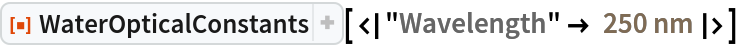Out=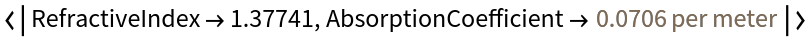Or temperature:

 In:=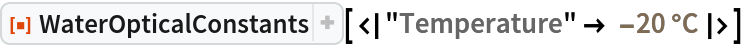Out=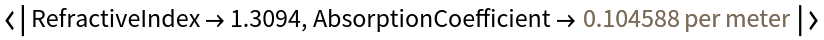### Scope (2)

If the units of the input are not specified, it is assumed to be in the default units:

 In:=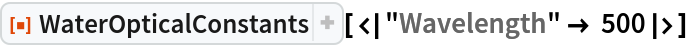Out=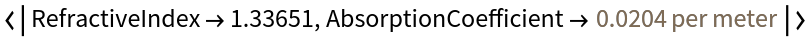Find the optical constants given specified values:

 In:=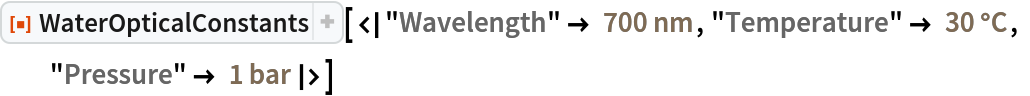Out=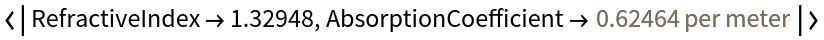### Properties and Relations (1)

Use Quantity to specify the units of the inputs:

 In:=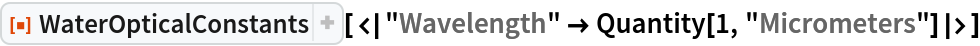Out=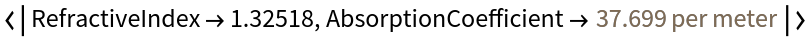### Possible Issues (1)

When the given wavelength is out of range for one of the optical constants, the value will be Missing:

 In:=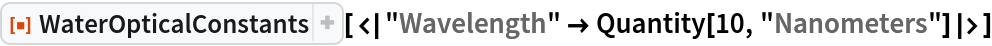Out=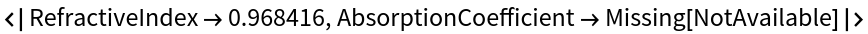### Neat Examples (2)

The function can be supplied to any suitable visualization function:

 In:=Out=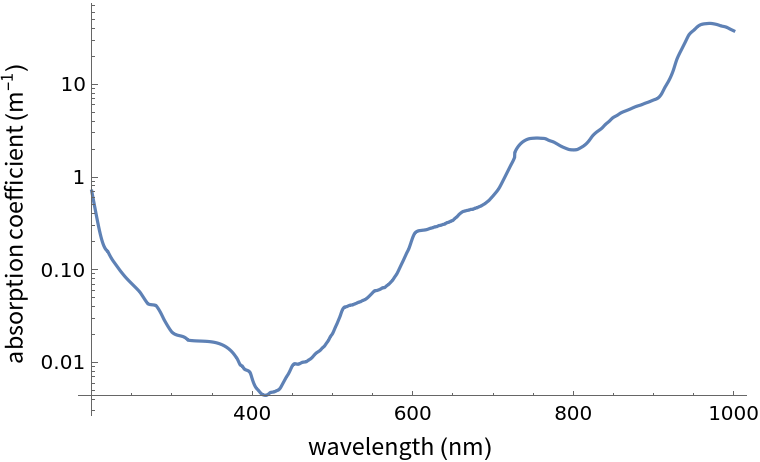Find the color of the wavelength with the minimum absorption coefficient in the visible spectrum:

 In:=Out=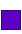## Publisher

WolframSpecialProjects

## Version History

• 1.0.0 – 21 December 2020